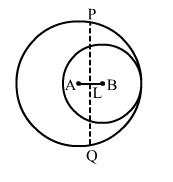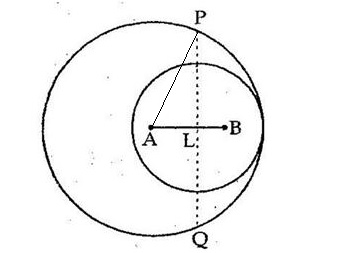# In the adjoining figure, two circles with centres at A and B, and of radii 5 cm and 3 cm touch each other internally.

Question:

In the adjoining figure, two circles with centres at A and B, and of radii 5 cm and 3 cm touch each other internally. If the perpendicular bisector of AB meets the bigger circle in P and Q, find the length of PQ.Solution:

Two circles with centres A and B of respective radii 5 cm and 3 cm touch each other internally.
The perpendicular bisector of AB meets the bigger circle at and Q.
Join AP.Let PQ intersect AB at point L.
Here, AP = 5 cm
Then AB = (5 - 3) cm = 2 cm
Since PQ is the perpendicular bisector of AB, we have:

$A L=\left(\frac{A B}{2}\right)=\left(\frac{2}{2}\right)=1 \mathrm{~cm}$

Now, in right angled ΔPLA, we have:

$A P^{2}=A L^{2}+P L^{2}$

$\Rightarrow P L^{2}=A P^{2}-A L^{2}$

$=5^{2}-1^{2}$

$=25-1=24$

$\Rightarrow \mathrm{PL}=\sqrt{24}=2 \sqrt{6} \mathrm{~cm}$

Thus PQ = 2 × PL

$=(2 \times 2 \sqrt{6})=4 \sqrt{6} \mathrm{~cm}$

Hence, the required length of $P Q$ is $4 \sqrt{6} \mathrm{~cm}$.